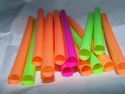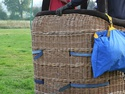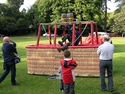## Working With Rational Numbers

Type of Unit: Concept

# Prior Knowledge

Students should be able to:

• Compare and order positive and negative numbers and place them on a number line.
• Understand the concepts of opposites absolute value.

# Lesson Flow

The unit begins with students using a balloon model to informally explore adding and subtracting integers. With the model, adding or removing heat represents adding or subtracting positive integers, and adding or removing weight represents adding or subtracting negative integers.

Students then move from the balloon model to a number line model for adding and subtracting integers, eventually extending the addition and subtraction rules from integers to all rational numbers. Number lines and multiplication patterns are used to find products of rational numbers. The relationship between multiplication and division is used to understand how to divide rational numbers. Properties of addition are briefly reviewed, then used to prove rules for addition, subtraction, multiplication, and division.

This unit includes problems with real-world contexts, formative assessment lessons, and Gallery problems.

Subject:
Mathematics, Algebra
Material Type:
Unit of Study
Level:
Middle School
7
Tags:
Fractions, Distance, Absolute Value, Integers, Number Line, Decimals, Addition, Rational Numbers, 7th Grade Mathematics, Subtraction, Multiplication, Problem-Solving, Expressions, Negative Numbers, Equations, Division
Unit 1 Working With Rational Numbers•••••••••••••••